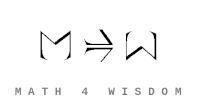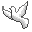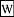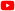Contact Andrius Kulikauskas ms@ms.lt +370 607 27 665 Eičiūnų km, Alytaus raj, LithuaniaMy original work is in the Public Domain for you to use in your best judgement. Support me! Patreon... in September? Paypal to ms@ms.ltBookshelfAs an Amazon Associate I earn from qualifying purchases. Thank you! Upload Schroedinger Equation Motivation I am trying to master the basics of Lie theory, and so I am developing my intuition by studying quantum physics. Schroedinger's equation is a key entry point, much like Newton's law {$F=ma$} but for quantum mechanics. In studying Griffith's book with my friend John Harland, I noticed the prominence of orthogonal polynomials in the solutions of Schroedinger's equation, and I realized that I could try to interpret them combinatorially. At this page, I overview my findings. Prerequisites In order to understand me, you should be familiar withSchrodinger's equation. I highly recommendIntroduction to quantum mechanics by Griffiths. Some encouragement in the search for hidden structure Maxwell's theory of the electromagnetic field, as we know, contains two hidden symmetries that will rock twentieth century physics. One is relativistic invariance and the other is gauge invariance. Now, both of these symmetries were already contained in Maxwell's theory, but nobody including Maxwell in the 19th century noticed it. And so is it conceivable that our present day theory also contains some kind of hidden structure? To me, that's a possibility. Hidden structure that's waiting for young people to discover just like Einstein discovered relativistic invariance hidden in Maxwell's equation and Hermann Weyl discovers gauge invariance in Maxwell's theory. – Anthony Zee, October 14, 2020,Quantum Field Theory: An Overview, 29:15. Combinatorial Interpretations of Solutions I'm exploring combinatorial themes that arise for the quantum oscillator but also other cases where the time independent Schroedinger's equation has nice solutions. Analytic symmetry The differential equation {$\frac{\textrm{d}}{\textrm{dx}}\psi=\psi$}, with solution {$\psi = Ce^x$}, is the simplest self-referential differential equation. As such it has a self-referential symmetry which I will call analytic symmetry. Mathematicians exploit this analytic symmetry to solve ever more sophisticated differential equations including the Schroedinger equation. Global constraints Schroedinger's equation is specified for a particular case by indicating the potential {$V(x)$}, which can be thought of as expressing the global constraints. For example, it can imply where necessarily {$\psi=0$}, and thus restrict the domain of the integrals on which we subsequently calculate probabilities. Examples... Mother function The global constraints, for example, that the behavior at infinity be physically acceptable, establishes the contours of the generally expected solution. In the case of the quantum oscillator, it means that the solutions {$\psi$} should not blow up as {$x$} grows infinite, but will tend towards {$e^{-x^2/2}$}, which makes sense for a second order differential equation. {$e^{-x^2/2}$} can be thought of as the mother function. It expresses the ground state solution, the behavior given minimal energy, and thus describes the background assumptions about space and time. Furthermore, other solutions will modify this mother function, which is to say, they will be multiples of it. There will be infinitely many such solutions, and they can all be thought of as arising from acting on the mother function with a raising operator. That operator combines multiplying by {$x$} and differentiating by {$\frac{\textrm{d}}{\textrm{dx}}$}, which expresses the contribution from momentum. Wrapper... Orthogonal polynomials The factors and their mother function satisfy a second order differential equation. This means that the factors are polynomials which satisfy a second order recurrence relation. In the cases of interest, they are orthogonal polynomials. For the quantum oscillator, they are Hermite polynomials. Perfect balance between position and momentum Position and momentum are two different quantities, which yields the slack so that there can be dynamics. But they need to perfectly balance each other so that the powers don't blow up going to infinity in either direction. The restrictions on this balance mean that we can have only finitely many nonzero coefficients in the polynomial solutions. Position vs. Momentum I looked at a proof of the orthogonality of the Hermite polynomials. It hinges on the lowering operator. We go from {$\textrm{He}_n$} to {$\textrm{He}_{n-1}$} by removing a cell from all terms. We go from {$\textrm{He}_m$} to {$\frac{\textrm{d}}{\textrm{dx}}\textrm{He}_m$} by removing, in each term, one of the empty cells. Here are my research notes: Research.SchroedingerEquation As usual, many of my observations are speculative. You may wonder, What does Andrius know about quantum physics?
Šis puslapis paskutinį kartą keistas March 09, 2021, at 11:38 AM